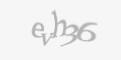0
0
0
s2sdefault

A quick little bash experiment to calculate some time.

I needed to calculate and verbalize some times elapsed. Here's the final experiment that finally got it going.

#!/bin/bash

ELAPSED=\$(( 3600 * \$(shuf -i 0-24 -n 1) ))

ELAPSED=\$(( \$ELAPSED + ( 60 * \$(shuf -i 0-24 -n 1) ) ))

ELAPSED=\$(( \$ELAPSED + \$(shuf -i 0-24 -n 1) ))

echo -n "Time: "

if [ "\$ELAPSED" -ge "3600" ]
then
echo -n "\$(( \$ELAPSED / 3660 )) hours, "
ELAPSED=\$(( \$ELAPSED - ( 3600 * (\$ELAPSED / 3600) ) ))
fi

if [ "\$ELAPSED" -ge "60" ]
then
echo -n "\$((\$ELAPSED / 60)) minutes and "
fi
echo "\$((\$ELAPSED % 60)) seconds!"